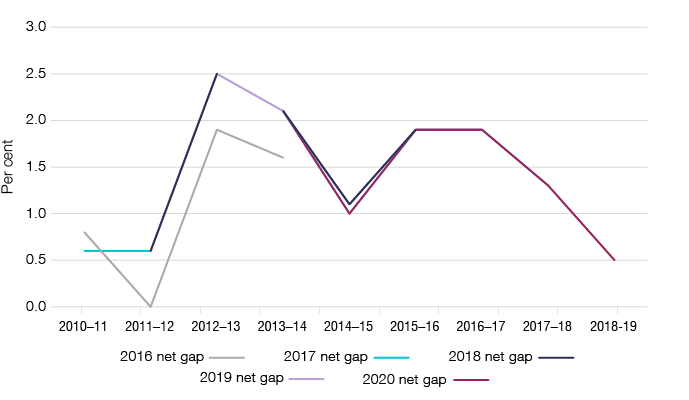• ## Methodology

The fuel excise tax gap estimate is derived through applying a top-down method. There are five steps in applying the top down methodology to estimate the fuel excise tax gap. These steps are explained below, followed by a summary of the overall estimate:

### Step 1: Estimate the total volume available

We estimate the excisable volume available in this order:

• estimate the volume imported
• add the volume produced in Australia
• remove the volume exported from Australia
• add the volume held in inventory at the end of the previous financial year
• remove the volume held in inventory at the end of the current financial year
• remove the volume not subject to excise.

### Step 2: Estimate average excise rates each year

Next, we estimate average excise rates.

### Step 3: Estimate theoretical excise liability amounts

We multiply the total volume available by the average excise rate to calculate the total theoretical liability excise liability.

### Step 4: Calculate the fuel excise tax net gap

We subtract ATO fuel excise revenue on an accrual basis using an economic transaction method (ETM) from the total theoretical excise liability to yield the net gap.

### Step 5: Calculate the fuel excise tax gross gap

We calculate the fuel excise tax gross gap by adding our compliance results to the net gap. Compliance outcomes are calculated by taking our compliance case results and allocating them to specific financial years on an accrual basis. The total excise compliance outcomes are apportioned using the percentage of total excise revenue attributable to petrol, diesel, ethanol, biodiesel and associated blends. We will review this assumption in future releases.

### Summary of the estimation process

Table 2 displays the methodology and dollar amounts for the steps used to estimate the fuel excise tax gap, and the percentage figures for the gross and net gaps.

Table 2: Applying the methodology – fuel excise tax gap

Step

Description

2013–14

2014–15

2015–16

2016–17

2017–18

2018–19

1 to 3

Total theoretical excise liability (\$m)

16,730

16,830

17,215

17,745

18,695

18,775

4.1

Less actual excise reported (\$m)

16,380

16,660

16,890

17,415

18,455

18,685

4.2

Equals fuel excise tax net gap (\$m)

350

170

325

330

240

90

5.1

0

10

0

0

1

0

5.2

Equals fuel excise tax gross gap (\$m)

350

180

325

330

241

90

5.3

Gross gap (%)

2.1

1.1

1.9

1.9

1.3

0.5

5.4

Net gap (%)

2.1

1.0

1.9

1.9

1.3

0.5

### Limitations

Although the gap methodology is reliable, it doesn’t account for variations due to temperature correction, timing and certain adjustments.

There could be errors stemming from non-standard measurement practices for the volumes of fuel products. Given that the volumes of fuel products would vary with temperature, they should always be measured at 15 degrees Celsius to maintain a consistent benchmark. However, Australian Petroleum Statistics volumes may be measured at different temperatures. Survey respondents are not given instructions to correct fuel volumes to 15 degrees Celsius.

A timing difference could arise between when product sales data are reported for compilation in the Australian Petroleum Statistics and when excise data enters ATO systems.

Assumptions around related volumetric calculations may also have an impact.

### Updates and revisions to previous estimates

While there has been no change to the methodology, updates to the underlying data have resulted in minor changes to previously published estimates.

Figure 2: Current and previous published net fuel excise tax gap estimates, 2010–11 to 2018–19The changes are set out in Table 2 and shown as a percentage.

Table 2: Current and previous published net fuel excise tax gap estimates (percentage), 2010–11 to 2018–19

Year published

2010–11

2011–12

2012–13

2013–14

2014–15

2015–16

2016–17

2017–18

2018–19

2016

0.8

0.0

1.9

1.6

n/a

n/a

n/a

n/a

n/a

2017

0.6

0.6

2.5

2.1

1.1

1.9

n/a

n/a

n/a

2018

n/a

0.6

2.5

2.1

1.1

1.9

1.9

n/a

n/a

2019

n/a

n/a

2.5

2.1

1.0

1.9

1.9

1.3

n/a

2020

n/a

n/a

n/a

2.1

1.0

1.9

1.9

1.3

0.5